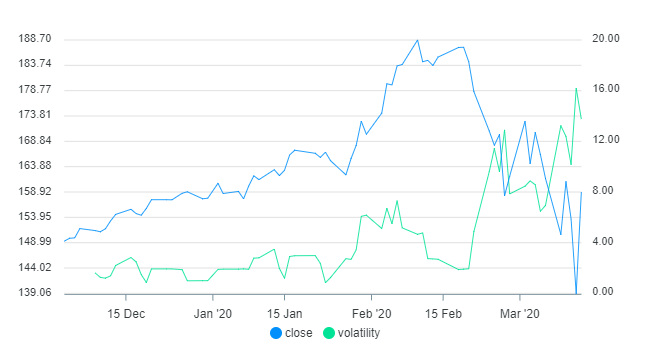Computing stock price volatility with Data-Forge

This blog post was exported from Data-Forge Notebook. You can edit and evaluate this notebook with Data-Forge Notebook.

This is an example JavaScript notebook that shows how to use the `percentRange` function from the Data-Forge code library to compute percent volatility for time series data.

In this post we are computing the 5-day volatility for Microsoft's stock price. This is particularly interesting because we can see how the recent plunge in the stock market has affected volatility of the stock.

## Install code libraries

If you are using Data-Forge Notebook simply requiring the libraries (as shown in the next section) means those libraries will be automatically installed. If you are using Node.js you'll have to install the code libraries manually as follows:

``npm install --save datakit data-forge dayjs``

## Import code libraries

Import the code libraries we need for this example:

``const datakit = require("datakit");const { DataFrame } = require("data-forge");const dayjs = require("dayjs");``

Load the data from a CSV file into a dataframe and preview the first 3 rows:

``const data =     await datakit.readCsv("./MSFT.csv", { // Load data.        parser: {             timestamp:                 v => dayjs(v, "YYYY-MM-DD").toDate(), // Parse dates.        },    }); let df = new DataFrame(data);   // Load data into dataframe.display(df.head(3));            // Preview our data.``
index timestamp open high low close volume SMA
0 2019-12-03 00:00:00 147.49 149.43 146.65 149.31 25192145 146.33500000000004
1 2019-12-04 00:00:00 150.14 150.1799 149.2 149.85 17580617 146.78433333333336
2 2019-12-05 00:00:00 150.05 150.32 149.48 149.93 17880601 147.20733333333337

## Compute volatility

Here we compute percentage volatility of the closing price over a 5-day period (how much % the stock has changed over the week):

``const volatility =    df.getSeries("close")       // Get the "close" series from the dataframe.        .percentRange(5)        // Compute % volatility with a 5-day period.        .round(2);              // Round to two decimal places.display(volatility.head(3));    // Preview computed volatility.``
index value
4 1.61
5 1.26
6 1.2

## Integrate volatility

Integrate the computed % volatility back into the dataframe:

``df = df.withSeries({ volatility }); // Integrate "volatility" column.display(df.skip(4).head(3));        // Preview integrated data.``
index timestamp open high low close volume SMA volatility
4 2019-12-09 00:00:00 151.07 152.21 150.91 151.36 16741350 147.95533333333333 1.61
5 2019-12-10 00:00:00 151.29 151.89 150.765 151.13 16481060 148.18666666666667 1.26
6 2019-12-11 00:00:00 151.54 151.87 150.33 151.7 18860001 148.48233333333334 1.2

## Plot chart

Plot the closing price against our computed % volatility. Notice how volatility increases quickly as the stock prices drops:

``display.plot(    df.toArray(),     {},     { x: "timestamp", y: "close", y2: "volatility" } );``## What can we do with this?

Volatility is used to assess how much a time series is fluctuating. You can use this in your trading strategy however you like. Does your strategy rely on highly volatile stocks? This is how you can measure that. Do you prefer to invest in less volatile stocks? This can do that as well.

Volatility is also a useful measure of potential risk and/or profit. The amount of movement in the past day, week or month gives you some indication of how far down (potential risk) or far up (potential profit) the stock might move if you held a position for that long. Better yet use a moving average of volatility to take the average of past volatility into account.

You might even use volatility as a market filter, only trading either if the market is highly volatile or less volatile, depending on the needs your strategy!

You can edit and evaluate this example notebook with Data-Forge Notebook